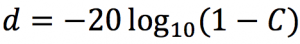# Decibel Dropp & Noise Reduction Coefficients For Material Combinations

Material manufacturers are required by law to publish Material Safety Data Specification (MSDS) sheets for their products. The MSDS sheet consists of the chemical and physical properties of a particular material like fiberglass or mineral wool. In addition to the MSDS, companies manufacturing materials that target the sound-reduction market often publish sound-reduction coefficients for their materials. The coefficients target individual frequencies. A problem arises when a company wants to offer a combination of materials. You just can’t add up the coefficients for the different materials. There is no formula you can use to determine the combination. The way to determine the coefficient is to test your combination of materials. In this article, we will take you through the testing process and show how the coefficients are calculated.

## The Sound Test

For example, our material combination may consist of a layer of one-inch fiberglass, two layers of ⅛-inch silent tread (recycled tires) and two layers of ⅛-inch EVA (mass loaded vinyl). We will need a sound room – pretend that you have constructed a soundproof room. Inside this room is a wall dividing the room in half. There is a 2-foot by 3-foot cutout in this dividing wall. On one side you have your sound source, and on the other side you have your audio analyzer hooked up to a laptop. You turn on your sound source at a certain frequency, 1 kHz, and measure the sound pressure level (SPL) in decibels (dB) coming through the opening from the source side. Let us say the SPL reading is 86.4 dB. This is your control value — 86.4 dB. Next, you fabricate a sound jacket. You fashion your sound jacket to the dividing wall covering the 2 by 3 foot hole in the wall. When you have it secured, you take another sound measurement and you find the reading is now 63.3 dB. The decibel drop is 23.1 dB.

To determine the coefficient (C) of sound reduction, use the decibel drop (d) in the following formula:Example: for a 23.1 dB drop, the coefficient is 0.93:Therefore, the coefficient for this material combination at 1 kHz is 0.93. This test will need to be repeated for different frequencies. Typically, these frequencies are 125 Hz, 250 Hz, 500 Hz, 1 kHz, 2 kHz, and 4 kHz. Once the tests are done at the different frequencies, a table like the following can by published.

 Frequency 125 Hz 250 Hz 500 Hz 1 kHz 2 kHz 4 kHz dB drop 19.4 21.6 22.0 23.1 22.9 22.1 coefficient .89 .92 .92 .93 .93 .92

## Using the Coefficient to Calculate Decibel Drop

To determine the decibel drop (d) with the material at the given frequency, we can use the coefficient (C) in this formula:Example: for a coefficient 0.78, the decibel drop is 13:## Conclusion

Published sound-reduction coefficients for a material are used to determine sound pressure level decibel drops. The coefficients and decibel drops are related by the mathematical formulas above. However, when combining different materials to reduce sound, the combined-materials product will need to be tested to determine its coefficients or decibel drop characteristics. Keep in mind the coefficients are for certain frequencies and it’s important that you understand which frequency or frequencies of sound you are trying to reduce.